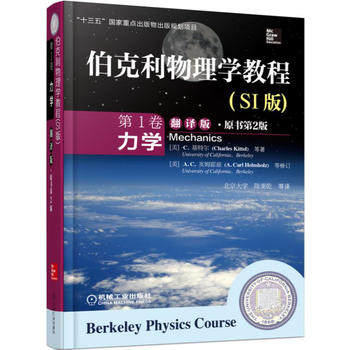## 一种测量表面张力的简单方法Eur. J. Phys.35(2014) 055003 介绍了一种简单方法。

- 阅读剩余部分 -

## 系绳球运动《伯克利物理学教程·力学》翻译版封面

《伯克利物理学教程·力学》第6章习题12：

(a) 瞬时转动中心在哪里？
(b) 绕通过柱中心的轴是否有力矩？角动量是否守恒？
(c) 设动能守恒，球转了5整圈之后，角速度是多少？- 阅读剩余部分 -

## 质点-哑铃碰撞《伯克利物理学教程·力学》翻译版封面

《伯克利物理学教程·力学》第6章习题11：

- 阅读剩余部分 -

## 长链落体《伯克利物理学教程·力学》翻译版封面

《伯克利物理学教程·力学》第6章习题8：

- 阅读剩余部分 -

## 质点碰撞损失多少动能？《伯克利物理学教程·力学》翻译版封面

《伯克利物理学教程·力学》第6章习题10：

- 阅读剩余部分 -

## 摩擦对于卫星运动的影响《伯克利物理学教程·力学》翻译版封面

《伯克利物理学教程·力学》第6章习题2：

- 阅读剩余部分 -

## 质心参考系中处理两个粒子的弹性碰撞《伯克利物理学教程·力学》翻译版封面\begin{equation} M_1v_1=M_1v'_1\cos\theta_1+M_2v'_2\cos\theta_2 \label{momentumconsx} \end{equation}

\begin{equation} 0=M_1v'_1\sin\theta_1+M_2v'_2\sin\theta_2 \label{momentumconsy} \end{equation}

\begin{equation} \frac{1}{2}M_1v_1^2=\frac{1}{2}M_1{v'}_1^2 + \frac{1}{2}M_2{v'}_2^2 \label{energycons} \end{equation}

- 阅读剩余部分 -

## 波的动图## 推导惯性系之间的坐标变换- 阅读剩余部分 -

## 由伽利略不变性推导动量守恒定律- 阅读剩余部分 -